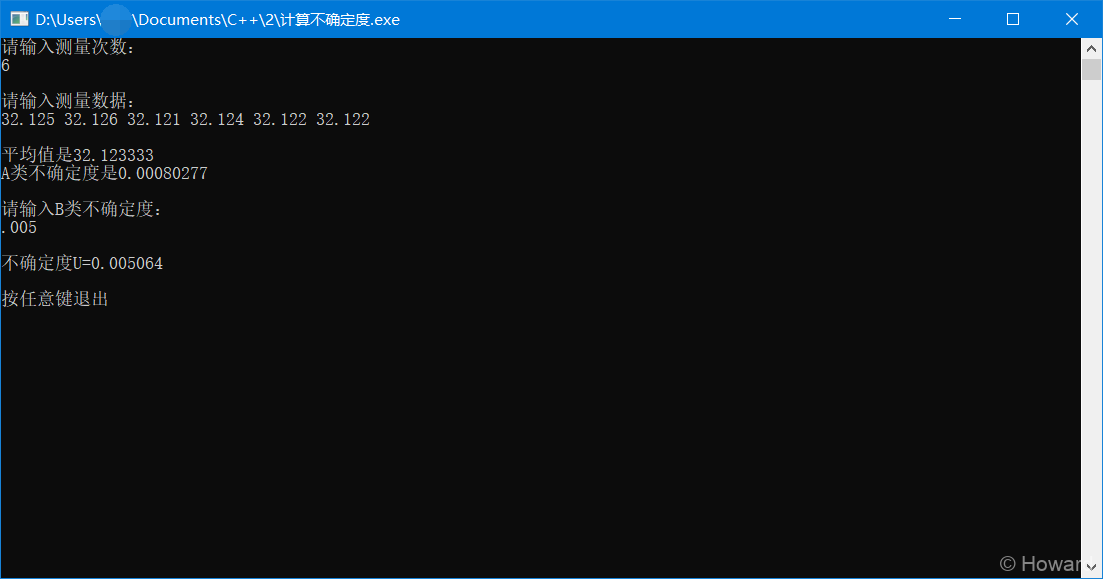# 一个计算不确定度的小程序

### 运行效果

##### 点击展开图片### 代码如下

##### C语言源代码
``````
/*
20200317
*/
#include <stdio.h>
#include <conio.h>
#include <math.h>
#define N 100
//粘贴过来后代码缩进没了，凑合看吧
int main()
{
int i,n=0;
double in[N];
double avd=0, Ua=0,Ub=0,U,sum=0,sum2=0;
printf("请输入测量次数：\n");
scanf("%d",&n);
printf("\n请输入测量数据：\n");
for(i=0;i<n;i++){
scanf("%lf",&in[i]);
sum+=in[i];
}
avd=sum/n;
for(i=0;i<n;i++){
sum2+=pow((in[i]-avd),2);
}
Ua=sqrt((sum2)/(n*(n-1)));
printf("\n平均值是%lf\nA类不确定度是%.8lf\n",avd,Ua);//A类不确定度保留8位有效数字，需要的自己调
printf("\n请输入B类不确定度：\n");
scanf("%lf",&Ub);
U=sqrt(pow(Ua,2)+pow(Ub,2));
printf("\n不确定度U=%lf\n",U);
printf("\n按任意键退出");
while(!kbhit());
return 0;
}``````